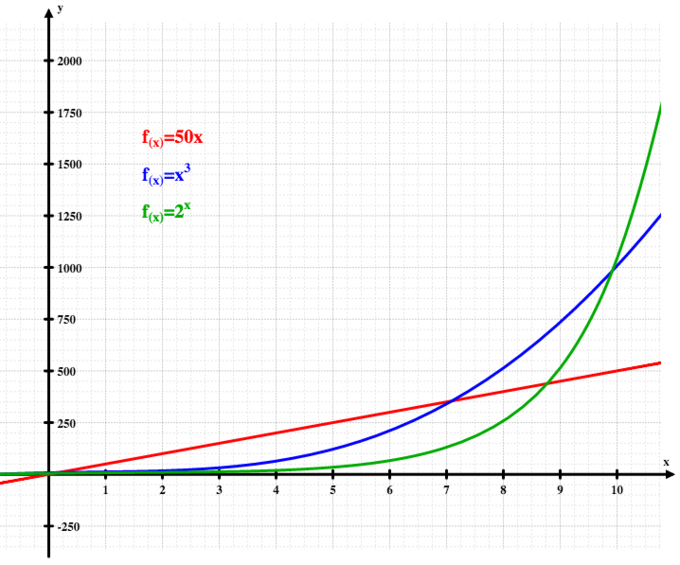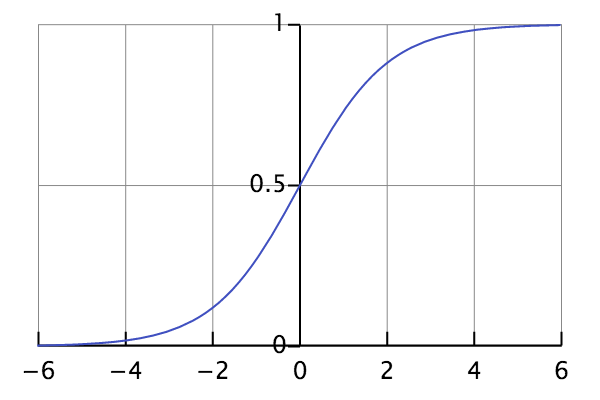## Four Ways to Represent a Function

Functions can be expressed in four different ways.

### Learning Objectives

Express a function verbally, algebraically, numerically, and graphically

### Key Takeaways

#### Key Points

• A function can be represented verbally. For example, the circumference of a square is four times one of its sides.
• A function can be represented algebraically. For example, $3x+6$.
• A function can be represented numerically.
• A function can be represented graphically.

#### Key Terms

• function: a relation in which each element of the domain is associated with exactly one element of the co-domain

A function is a relation between a set of inputs and a set of permissible outputs, provided that each input is related to exactly one output. An example is the function that relates each real number $x$ to its square $x^2$. The output of a function $f$ corresponding to an input $x$ is denoted by $f(x)$ (read “f of x“). In this example, if the input is $-3$, then the output is 9, and we may write $f(-3)=9$. The input variable (s) are sometimes referred to as the argument(s) of the function.

Modern calculus texts emphasize that a function can be expressed in four different ways.

Verbal: When modeling a process mathematically, one often first develops a verbal description of the problem. For example, the expression $2x+6$ can be represented as “Double x and add six” or “six added to two times x”.

Algebraic: This is the most common, most concise, and most powerful representation: $2x+6$. Note that in an algebraic representation, the input number is represented as a variable (in this case, an x).

Numerical: This can be expressed as a list of value pairs, as in $(4,14)$ — meaning that if a 4 goes in, a 14 comes out. (You may recognize this as the $(x,y)$ points used in graphing.)

Graphical: This involves modeling a function in a dimensional overlay. Scientific data is often recorded in a visual format. Examples include seismograph readings, electrocardiograms, and oscilloscope readings.

These are not four different types of functions; they are four different views of the same function. One of the most important skills in algebra and calculus is being able to convert a function between these different forms, and this theme will recur in different forms throughout the text.

## Essential Functions for Mathematical Modeling

Mathematical models are used to explain systems, study effects of components, and make predictions about behavior.

### Learning Objectives

Discuss the uses of mathematical models

### Key Takeaways

#### Key Points

• A mathematical model is a description of a system using mathematical concepts and language.
• Mathematical models are used in the natural sciences, engineering disciplines, and in the social sciences.
• Mathematical models can take many forms, including but not limited to dynamical systems, statistical models, differential equations, or game theoretic models.

#### Key Terms

• map projection: any systematic method of transforming the spherical representation of parallels, meridians and geographic features of the Earth’s surface to a nonspherical surface, usually a plane
• exponential growth: The growth in the value of a quantity, in which the rate of growth is proportional to the instantaneous value of the quantity; for example, when the value has doubled, the rate of increase will also have doubled. The rate may be positive or negative.
• mathematical model: An abstract mathematical representation of a process, device or concept; it uses a number of variables to represent inputs, outputs and internal states, and sets of equations and inequalities to describe their interaction.

A mathematical model is a description of a system using mathematical concepts and language. The process of developing a mathematical model is termed mathematical modeling. Mathematical models are used not only in the natural sciences (such as physics, biology, earth science, meteorology) and engineering disciplines (e.g. computer science, artificial intelligence), but also in the social sciences (such as economics, psychology, sociology and political science); physicists, engineers, statisticians, operations research analysts and economists use mathematical models most extensively. A model may help to explain a system and to study the effects of different components, and to make predictions about behavior.

Mathematical models can take many forms, including but not limited to dynamical systems, statistical models, differential equations, or game theoretic models. These and other types of models can overlap, with a given model involving a variety of abstract structures. In general, mathematical models may include logical models, as far as logic is taken as a part of mathematics. In many cases, the quality of a scientific field depends on how well the mathematical models developed on the theoretical side agree with results of repeatable experiments. Lack of agreement between theoretical mathematical models and experimental measurements often leads to important advances as better theories are developed.

For example, a simple model of population growth is the Malthusian growth model. This is essentially exponential growth based on a constant rate of compound interest: $P(t)=P_0e^{rt}$ where $P_0=P(0)=\text{initial population}$, $r$ is the growth rate, and $t$ is the time.Exponential Growth: The graph illustrates how exponential growth (green) surpasses both linear (red) and cubic (blue) growth.

A slightly more realistic and largely used population growth model is the logistic function that may be defined by the formula: $P(t) = \frac{1}{1 + e^{-t}}$.Logistic Curve: The standard logistic curve.

Notice the difference between this model and the previous. In the previous, the model predicts continued increase of population with time, whereas this more realistic model shows a decrease in growth rate until the population reaches an approximate steady state. Such a trend aligns with physical reality; there is a limit on the number of people finite environmental resources can sustain. Another example is a model of a particle in a potential-field. In this model we consider a particle as being a point of mass following a trajectory in space modeled by a function giving its coordinates in space as a function of time. The potential field is given by a function $V:R^3 \rightarrow R$ and the trajectory is a solution of a differential equation.

Even many everyday activities carried out without a thought are uses of mathematical models. A geographical map projection of a region of the earth onto a small, plane surface is a model which can be used for many purposes such as planning travel.